# Expression of a variable from the formula - 6th grade (11y) - math problems

#### Number of problems found: 140

• The expressionWhat is the value of the expression ((62+60))/(23)
• When 5When 5 is subtracted from both my numerator and denominator I become 3/4. What fraction i am?
• Evaluate expr with 2 varsIf a=6 and x=2, find the value of (2ax + 7x- 10) /(4ax-3a-2)
• Perimeter to area 2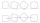Find the area of a square whose perimeter is 100 cm with solve.
• If x=4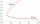If x=4 and y=-3, what is the value of the expression x² + 2xy - 4x + 3y + y²?
• A rectangular 3A rectangular plot of land has an area of 3/2 square kilometers and a length of ¾ kilometer.  What is the width of the plot of land?
• Maya is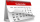Maya is 14 years old. Her brother Jorge is 3 years more than half her age. Which of the following is the correct expression for Jorge's age?
• Distributive propertyVerify the distributive property a×(b+c)=(a×b)+(a*c) for the rational number a=5/8, b=7/4 and c=2/3
• Tank B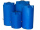Tank B has the same volume as Tank A. The volume of one section of Tank B is 4545 cubic feet. What is the volume, in cubic feet, of the other section of Tank B? Total volume of both tanks A+B is 15000 ft3. Enter your answer in the box.
• The two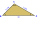The two angles of a triangle are 78° and 82°. So what is the measure of the remaining third angle?
• Two numbers and its productThe product of two numbers are 2/3. If on of them is 1/10, what is the other?
• A dumpster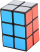A dumpster is shaped like a rectangular prism. It has a width of 8 feet, a height of 5 feet, and a volume of 880 cubic feet. What is the length of the dumpster?
• Cheese consumption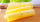I have some cheese. I used 2/5 of the total amount of cheese yesterday. Today, I used 2/5 of the remaining cheese from yesterday. If 900 g of cheese remain at the end of today, how many grams of cheese did I have originally?
• Edges of the cuboid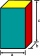Find the length of the edges of the cuboid, which has the following dimensions: width is 0.4 m; the height is 5.8 dm and the block can hold 81.2 liters of fluid.
• Evaluate 9Evaluate expression: 3 { 4 − 4 ( 5 − 12 ) } + 20
• Scrapbook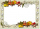Jo is making a scrapbook for her best friend’s 5th grade graduation. She used 72 photos with 6 photos on each page. Jo had to remove two of the photos on each page in order to make room for the writing she wanted to include. Write an expression represents
• Expression with bracketsEvaluate the expression. 15+[(9-4)×22]
• Cylinder container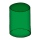If the cylinder-shaped container is filled with water to a height of 5 dm, it contains 62.8 hectoliters of water. Calculate the diameter of the bottom of the container. Use the value π = 3.14.
• Evaluate expressionEvaluate following expression: 5 to the power of 2 - (4x4) divided by 2 =
• Main/central vertex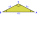ABC is an isosceles triangle with base BC and main vertex A. The angle at vertex A is 18°. What will be the size of the angle at vertex B?

Do you have an exciting math question or word problem that you can't solve? Ask a question or post a math problem, and we can try to solve it.

We will send a solution to your e-mail address. Solved examples are also published here. Please enter the e-mail correctly and check whether you don't have a full mailbox.

Expression of a variable from the formula - math problems. Examples for 6th grade (the sixth graders).